# Beams and Trusses

Source: Saylor

Student Price: Free with Top Hat Pro Subscription

A question pack on engineering mechanics.

Beams and Trusses Q29

Which of the following is true for statically indeterminate trusses?

I. They are inherently unstable.

II. They deflect more than statically determinate trusses.

III. Consideration of deflection is necessary to solve for all internal forces.

IV. They require welded joints rather than pin-connected joints.

A

II and IV only

B

IV only

C

II and III only

D

III and IV

E

III only

Beams and Trusses Q28Consider the structure depicted in figure below. There are two forces of 200kN and 100kN acting on point A. What is the tension in the segment AB?

A

‑150 kN

B

200 kN

C

‑200 kN

D

100 kN

E

‑100 kN

Beams and Trusses Q27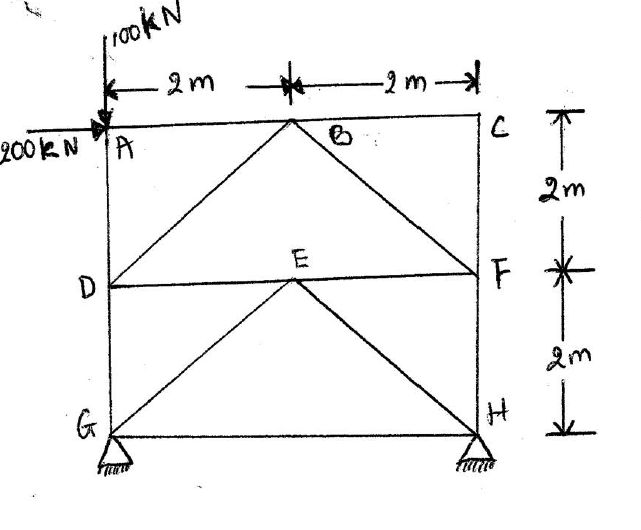Consider the structure depicted in figure below. There are two forces of 200kN and 100kN acting on point A. What is the tension in the segment AD?

A

‑150 kN

B

200 kN

C

‑200 kN

D

‑100 kN

E

‑50 kN

Beams and Trusses Q26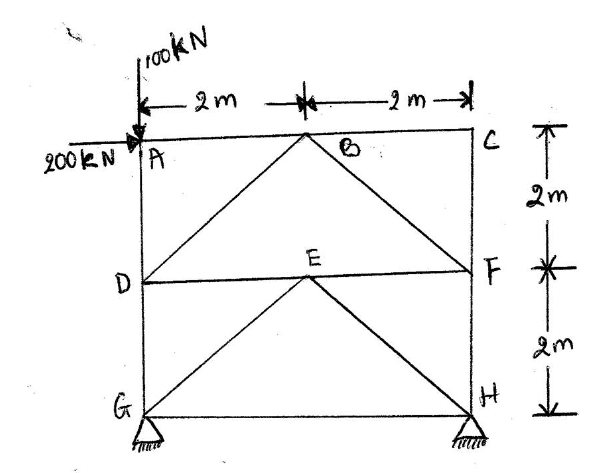Consider the structure depicted in the figure below. There are two forces of 200kN and 100kN acting on point A. What is the tension for the segment BC?

A

0 kN

B

50 kN

C

144 kN

D

‑50 kN

E

‑144 kN

Beams and Trusses Q25

Consider the structure depicted in figure below. There are two forces of 200kN and 100kN acting on point A. The distances between A and B as well as B and C are 2 m. What is the tension in the segment CF?

A

0 kN

B

50 kN

C

144 kN

D

‑50 kN

E

‑100 kN

Beams and Trusses Q24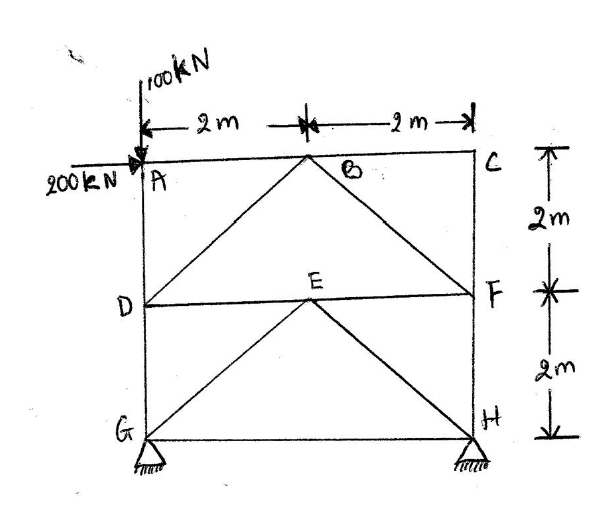Consider the structure depicted in figure below. There are two forces of 200kN and 100kN acting on point A. The distances between A and B as well as B and C are 2 m. What is the tension in the segment BD?

A

0 kN

B

50 kN

C

144 kN

D

‑50 kN

E

‑100 kN

Beams and Trusses Q23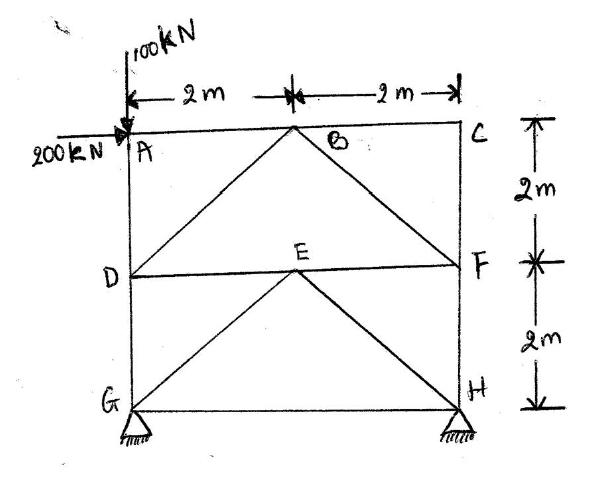Consider the structure depicted in the figure below. There are two forces of 200kN and 100kN acting on point A. The distances between A and B as well as B and C are 2 m. What is the tension in segment BF?

A

‑144 kN

B

50 kN

C

144 kN

D

‑50 kN

E

100 kN

Beams and Trusses Q22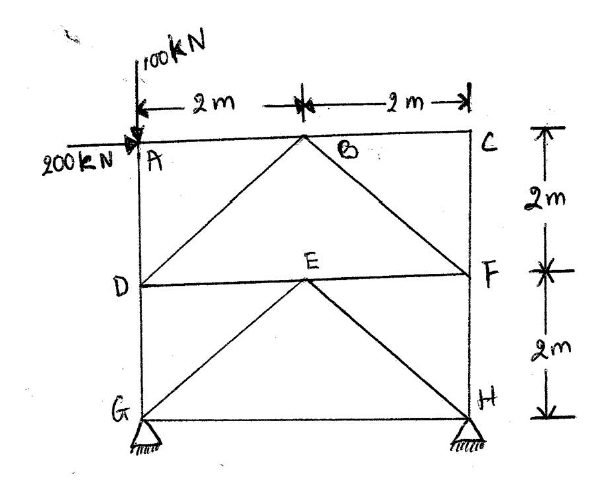Consider the structure depicted in figure below. There are two forces of 200kN and 100kN acting on point A. The distances between A and B as well as B and C are 2 m. What is the tension in the segment EG?

A

‑144 kN

B

50 kN

C

144 kN

D

‑50 kN

E

‑100 kN

Beams and Trusses Q21

How many members does a perfect truss with 8 joints have?

A

16

B

8

C

12

D

13

E

6

Beams and Trusses Q20

The number of unknowns at a joint should not exceed ______________, so the method of joints can be used to solve a planar truss problem.

A

4

B

3

C

2

D

1

E

9

Beams and Trusses Q19

Which of the following statements is true for a statically indeterminate beam?

A

The number of reactions is unknown.

B

The number of reactions is greater than the number of equilibrium equations.

C

The number of reactions is equal to the number of equilibrium relations.

D

The number of reactions is less than the number of equilbirium relations.

E

The number of reactions is equal to the number of equilibrium relations minus 2.

Beams and Trusses Q18

The deflection of a center loaded beam is approximately FL$^3$/48EI. For a beam with square cross section, I = b$^4$/12 (where b is the length of a side). If the deflection is 5 cm, b is 4 inches, L = 10 ft, and the load is 5kN, then what is the approximate value of Young’s modulus?

A

6.6 GPa

B

42 GPa

C

0.42 GPa

D

66 MPa

E

33 MPa

Beams and Trusses Q17

The deflection of a center loaded beam is approximately FL$^3$/48EI. For a beam with square cross section, I = b$^4$/12 (where b is the length of a side). If the deflection is 5 cm, b is 4 inches, L = 10 ft, and E = 20 GPa, then what is the load?

A

25 kN

B

15 kN

C

11 kN

D

9kN

E

90 kN

Beams and Trusses Q16

The deflection of a center loaded beam is approximately FLsup>3/48EI. For a beam with square cross section, I = b$^4$/12 (where b is the length of a side). If the load is 50 kN, b is 4 inches, L = 10 ft, and E = 20 GPa, then what is the deflection of the beam?

A

1 cm

B

3 cm

C

7 cm

D

12 cm

E

120 cm

Beams and Trusses Q15

The downward deflection of an end loaded cantilevered beam can be written as FL$^3$/3EI, where I is the area moment of inertia of the beam. How does the defection of the beam increase if the length is tripled and all else remains constant?

A

Increases by 6 fold

B

Increases by 9 fold

C

Increases by 3 fold

D

Increases by 81 fold

E

Increases by 27 fold

Beams and Trusses Q14

Which of the following best represents a simplified beam equation?

A

EI d $^4$/dx$^4$ = q(x)

B

EI d$^2$/dx$^2$= q(x)

C

EI d$^/$$^d$$^x$ $^=$$^q$$^x$

D

EIx d $^3$/dx$^3$ = q(x)

E

EI d$^3$/dx$^3$= q(x)

Beams and Trusses Q13

What form does a beam displacement equation typically take?

A

A constant coeffcient second order differential equation

B

A first order differential equation

C

A fifth order differential equation

D

A fourth order differential equation

E

A second order differential equation

Beams and Trusses Q12

To whom is the development of beam theory commonly attributed?

I. Bernoulli

II. Gauss

III. Tesla

IV. Liebnitz

V. Franklin

VI. Euler

A

I and VI

B

I, II, III, IV, and VI

C

I, II, and VI

D

V

E

IV and I

Beams and Trusses Q11

A horizontal beam simply supported at either end and loaded in the middle will exhibit which of the following?

A

Compression in the upper portion of the beam and tension in the lower portion_a

B

Compression in the upper portion of the beam and compression in the lower portion

C

Tension in the upper portion of the beam and compression in the lower portion

D

Compression in the upper portion of the beam and tension in the lower portion_b

E

Deflection or bending without tension or compression

Beams and Trusses Q10

How does a cantilever support differ from a simple beam support?

A

They do not differ.

B

A cantilever support can provide both support force and moment, whereas a simple support can only force at a point.

C

A simple support can provide both support force and moment, whereas a cantilever support can only force at a point.

D

A cantilever support can only provide transverse support, whereas a simple support can provide both vertical and horizontal support.

E

A cantilever support can provide only vertical support, whereas a simple support can only provide transverse support.

Beams and Trusses Q9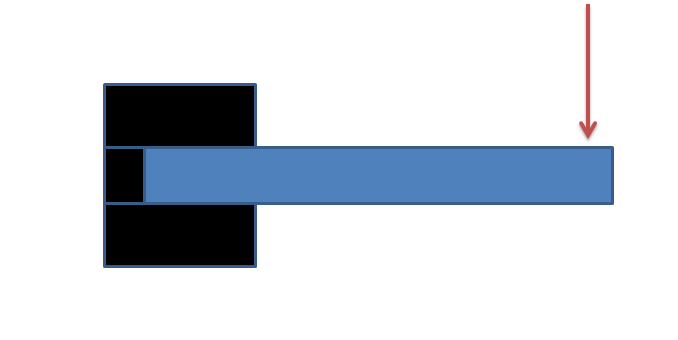Which of the following would be the most appropriate description of the beam configuration shown in the figure below?

A

B

C

Simply supported, cantilevered beam

D

E

Beams and Trusses Q8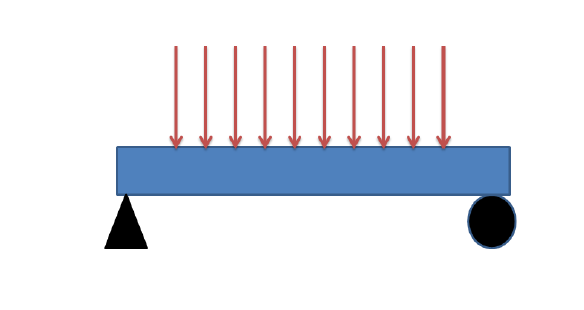Which of the following would be the most appropriate description of the beam configuration shown in the figure below?

A

B

Simply supported beam with a distributed load

C

Simply supported, cantilevered beam

D

E

Beams and Trusses Q7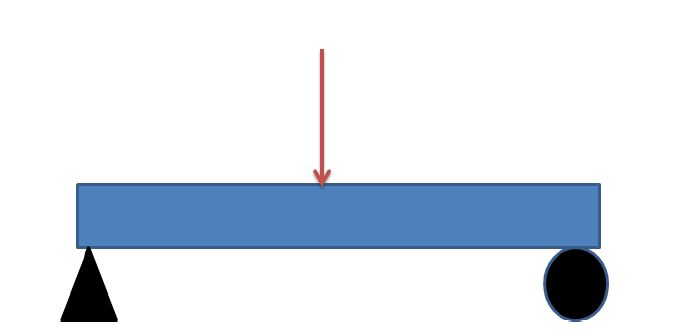Which of the following would be the most appropriate description of the beam configuration shown in the figure below?

A

B

Simply supported beam with a distributed load

C

Simply supported, cantilevered beam

D

E

Beams and Trusses Q6

A

Distributed loads occur over a range in space; discrete loads occur at specific locations.

B

Discrete loads occur over a range in space; distributed loads occur at specific locations.

C

There is no real difference.

D

Discrete loads may only have values in multiples of 2; distributed loads may have any value.

E

Distributed loads are evenly spaced; discrete loads may occur at any location.

Beams and Trusses Q5

How does a truss element differ from a beam?

A

Truss elements may only support axial loads; beams may support transverse loads.

B

They are the same.

C

Beams have complex cross sections; truss elements are rods.

D

Truss elements buckle under compression; beams do not do so as easily.

E

Beams do not support tension; truss elements do.

Beams and Trusses Q4

For a uniformly loaded cantilevered beam, how does shear within the beam depend upon position along the length of the beam?

A

It is constant.

B

It decreases linearly from the free end to the supported end.

C

It increases linearly from the free end to the supported end.

D

It increases quadratically from the free end to the supported end.

E

It is zero.

Beams and Trusses Q3

For an end‑loaded cantilevered beam, how does the bending moment depend upon position along the length of the beam?

A

It increases linearly from the load end to the cantilevered end.

B

It decreases linearly from the load end to the cantilevered end.

C

It is constant.

D

E

Beams and Trusses Q2

For an end‑loaded cantilevered beam, how does shear within the beam depend upon position along the length of the beam?

A

It is constant.

B

It decreases linearly from the free end to the supported end._a

C

It decreases linearly from the free end to the supported end._b

D

It increases quadratically from the free end to the supported end.

E

It is zero.

Beams and Trusses Q1

For a uniformly loaded cantilevered beam, how does the bending moment depend upon position along the length of the beam?

A

It increases linearly from the load end to the cantilevered end.

B

It decreases linearly from the load end to the cantilevered end.

C

It is constant.

D